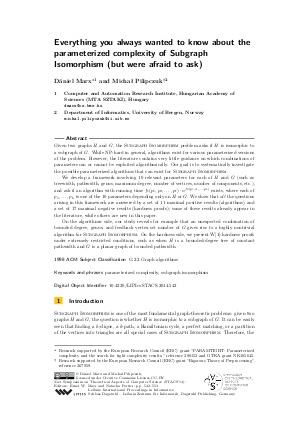Document# Everything you always wanted to know about the parameterized complexity of Subgraph Isomorphism (but were afraid to ask)

### Authors Dániel Marx, Michal Pilipczuk## File

LIPIcs.STACS.2014.542.pdf
• Filesize: 0.66 MB
• 12 pages

## Cite As

Dániel Marx and Michal Pilipczuk. Everything you always wanted to know about the parameterized complexity of Subgraph Isomorphism (but were afraid to ask). In 31st International Symposium on Theoretical Aspects of Computer Science (STACS 2014). Leibniz International Proceedings in Informatics (LIPIcs), Volume 25, pp. 542-553, Schloss Dagstuhl - Leibniz-Zentrum für Informatik (2014)
https://doi.org/10.4230/LIPIcs.STACS.2014.542

## Abstract

Given two graphs H and G, the Subgraph Isomorphism problem asks if H is isomorphic to a subgraph of G. While NP-hard in general, algorithms exist for various parameterized versions of the problem. However, the literature contains very little guidance on which combinations of parameters can or cannot be exploited algorithmically. Our goal is to systematically investigate the possible parameterized algorithms that can exist for Subgraph Isomorphism. We develop a framework involving 10 relevant parameters for each of H and G (such as treewidth, pathwidth, genus, maximum degree, number of vertices, number of components, etc.), and ask if an algorithm with running time f1_(p_1,p_2,...,p_l).n^f_2(p_(l+1),...,p_k) exists, where each of p_1,...,p_k is one of the 10 parameters depending only on H or G. We show that all the questions arising in this framework are answered by a set of 11 maximal positive results (algorithms) and a set of 17 maximal negative results (hardness proofs); some of these results already appear in the literature, while others are new in this paper. On the algorithmic side, our study reveals for example that an unexpected combination of bounded degree, genus, and feedback vertex set number of G gives rise to a highly nontrivial algorithm for Subgraph Isomorphism. On the hardness side, we present W-hardness proofs under extremely restricted conditions, such as when H is a bounded-degree tree of constant pathwidth and G is a planar graph of bounded pathwidth.
##### Keywords
• parameterized complexity
• subgraph isomorphism

## Metrics

• Access Statistics
• Total Accesses (updated on a weekly basis)
0